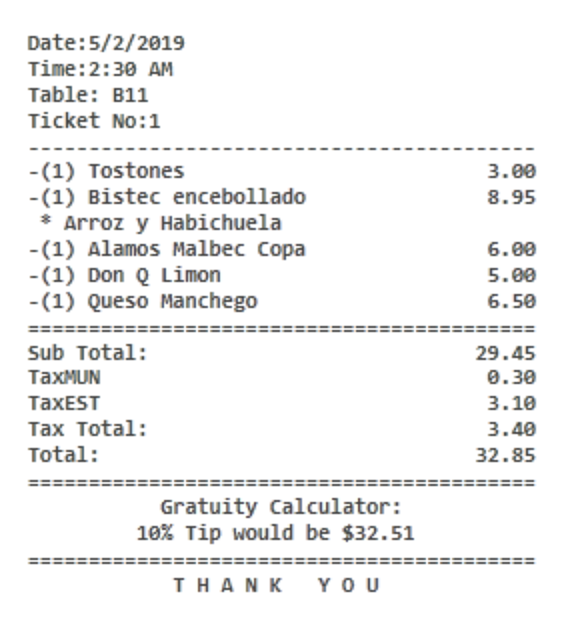# Gratuity / Tip Calculator on Bill

Hiya,

I’ve made a tip calculator printed on bills from a script QMcKay gave me for my tax amount on my bills.
It is a great way to help promote tipping for our staff. Try it out if it suits your establishment.

`<F>= <EB> <C00>Gratuity Calculator: <DB> <C00>10% Tip would be R[=F(TN('{TICKET TOTAL}') * 0.10)] <C00>15% Tip would be R[=F(TN('{TICKET TOTAL}') * 0.15)] <F>=`

6 Likes

Now a question… if I wanted the tip calculator to only show a rounded figure to the nearest whole number…

`[=F(TN('{TICKET TOTAL}') * 0.15)]`

e.g R1,60 should be --> R2
and R2,40 should be --> R2

(for those inquisitive, R symbol stands for RANDS it’s the currency of South Africa. 15 Rand = 1 Euro)

Thanks QMcKay

Try this?

``````[=Math.round(TN('{TICKET TOTAL}') * 0.15)]
``````
1 Like

No sorry that left it blank

Try this
`[=F(TN('{TICKET TOTAL}') * 0.15 +0.5, '0')]`

1 Like

Sorry, try again - I edited my post, should be lowercase ‘r’ on the “round”…

``````[=Math.round(TN('{TICKET TOTAL}') * 0.15)]
``````
1 Like

Yes works beautifully !

`[=Math.round(TN('{TICKET TOTAL}') * 0.15)]`

Thank you, and to you too Sukasem for the effort

Hi guys
How did you make the TiP:_________ show on the ticket??

Add the line on the ticket template…

Thanks For this code !!

But I Want to calculate only with the sub-total I am trying this code

`<C00> 10% Tip would be \$[=F(TN('{TICKET TOTAL}')-TN('{TAX TOTAL}') * 0.10)]`

And I Don’t Know what calculate… Can you help me

• I only want to Calculate the tip with the sub total!Use PLAIN TOTAL in your calculation.

`\$[=F(TN('{PLAIN TOTAL}') * 0.10)]`

2 Likes